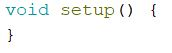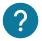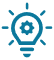# What is the void setup? What instructions we have to write in it?

The void setup is a function created at the beginning of the program, between the initialization of the variable and the void loop. The void setup contains the initialization of the components such as an input or an output of the Arduino card, an initialization of the serial monitor. We only initialize the components that we want to control.

The void setup is a function which will execute only one time at the beginning of the program.

``````void setup(){
}``````### Don't forget the void setup!

The void setup function is obligatory in every Arduino programs, even if there isn’t anything inside. Forget to write it will create an error.### What can we write in this function?

In this function you can initialize a value to a variable, assign a component to a pin and import a library or the serial monitor.

Here is an example of what we can write in the void setup:

``````void setup(){
Serial.begin(9600); // Initialization of the serial monitor at 9600 bit/s or bauds.
Serial.println("Decoding"); // To write a message in the serial monitor at the beginning of the program and juste one time.
pinMode(2, OUTPUT); // Initializing the pin 2 as an output
pinMode(5, INPUT) // Initializing the pin 2 as an input
pinMode(LED, OUTPUT) // Initializing the led as an output
}
``````

As we can see in this example there are many instructions:

• Serial.begin(9600): To initialize the serial monitor that we will use to display message or values.
• Serial.println(“Decoding”): To display “Decoding” in the serial monitor.
• pinMode(2,OUTPUT):  To initialize the pin as an output to control a component.
• pinMode(5,INPUT): To initialize the pin as an input to retrieve the state value of a push button for example.
• pinMode(LED,OUTPUT): To initialize the LED as an output. To do that you have to initialize the variable LED (int LED =4;) before calling it in the void setup.### Advantage of the void setup

We will ask us in this part what is the advantage of the void setup compared to the void loop?

The most important advantage of the void setup is that it’s only executed one time at the beginning. That way you can initialize variable and  the serial monitor.

Here is some ideas of what you can put inside:

• Thanks to the void setup you can write a message in the serial monitor and it will be displayed only once. It can be useful if you want to write “initialization of the program” for example
• Thanks to the void setup you can turn on a component at the beginning of the program, such a red led if you are doing a safety deposit box for example, or replace a servomotor at a initial position at the beginning of a program.